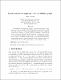## Linear and metric maps on trees via Markov graphs : [preprint]2018
##### Authors
Kozerenko, Sergiy
##### Abstract
The main focus of combinatorial dynamics is put on the structure of periodic points (and the corresponding orbits) of topological dynamical systems. The first result in this area is the famous Sharkovsky's theorem which completely describes the coexistence of periods of periodic points for a continuous map from the closed unit interval to itself. One feature of this theorem is that it can be proved using digraphs of a special type (the so-called periodic graphs). In this paper we use Markov graphs (which are the natural generalization of periodic graphs in case of dynamical systems on trees) as a tool to study several classes of maps on trees. The emphasis is put on linear and metric maps.
##### Keywords
Markov graphs, Sharkovsky's theorem, maps on trees, preprint, article
##### Citation
Kozerenko S. Linear and metric maps on trees via Markov graphs / Sergiy Kozerenko // Commentationes Mathematicae Universitatis Carolinae. - 2018. - Vol. 59, Issue 2. - P. 173-187.